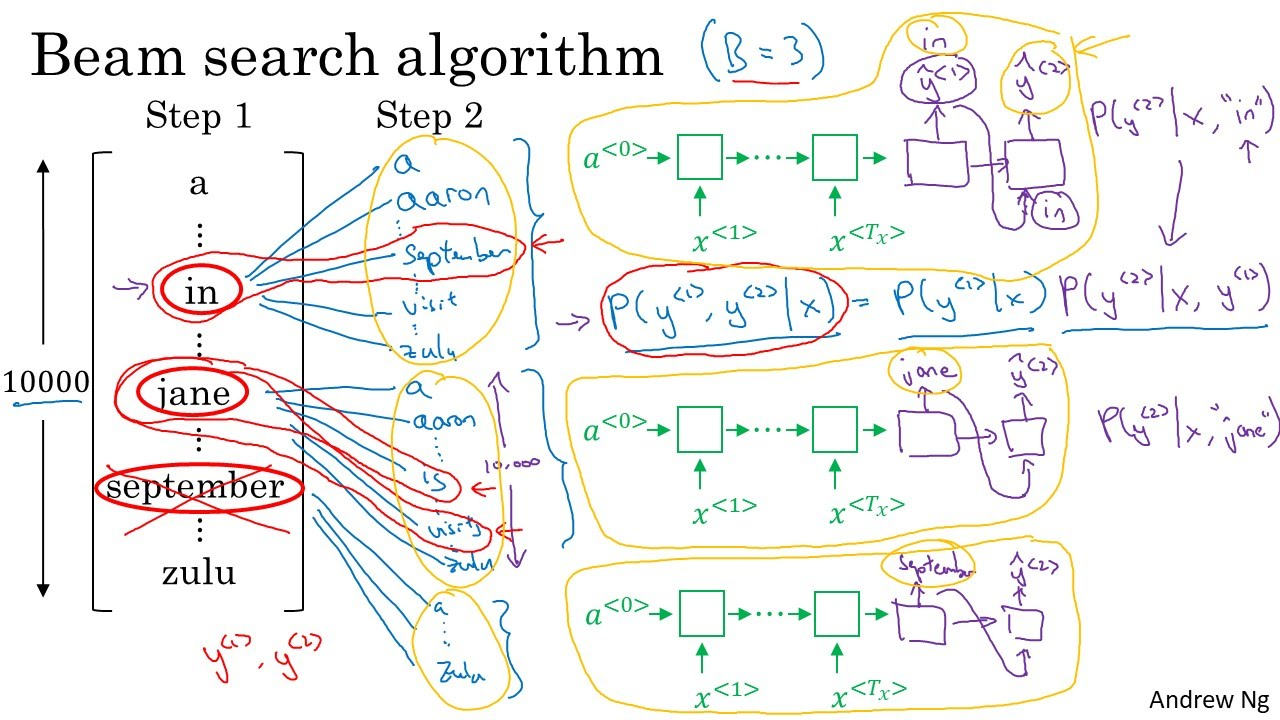# Beam Search

Categories:

Updated:

Beam search is used when picking likely words in the language model like part of sequence to sequence model.

# Why Beam Search?

If we just pick the most likely word from $\hat{y}^{<1>}$ , feed it into the next sequential layer, pick the most likely word from $\hat{y}^{<2>}$ , feed it into the next sequential layer,… it might not be the best choice; there might be other combinations of words that can maximize the probability.

We might try all combinations of words and select the most probable one. However, this takes too much computational cost.

To compromise from these two options, ‘Beam Search’ was invented.

# Beam Search Algorithm

Beam search algorithm is simple.

First, we set beam width $B$ . Then, for every sequential layer, pick $B$ most probable word and feed each of them into the next sequential layer. We repeat this until we meet <EOS>, and select the combination that has the highest probability.

Let’s look at an example.Let’s set beam width to 3. In the first step(first sequential layer), let’s say that the most probable three words are ‘in’, ‘jane’, and ‘september’. Then, we calculate $\hat{y}^{<2>}$ for each of these three cases $x^{<2>}=$ ‘in’, $x^{<2>}=$ ‘jane’, $x^{<2>}=$ ‘september’. Since the vocab size is 10000, we are searching from 30000 candidates. Again, select the three most probable words…

### 1. Scaling to Log

Our original objective of sequence to sequence model was;

However, when a computer multiplies too many small $p$ s, there might be errors. So we take log. Then our objective is as follows;

### 2. Length Normalization

If the output sequence length $T_y$ becomes bigger, the sum of the log proababilities becomes smaller. So we have to give handicap when $T_y$ is big. Now our objective becomes following;

where $\alpha$ is a hyperparmeter that controls how much we penalize short output sequences.

# Error Analysis on Beam Search

We look into the examples in dev set where our model failed. Then we diagnose if the error is due to the model itself or due to small beam width, by doing as follows;

1. $p(y|x) > p(\hat{y}|x)$ :Need to increase beam width in this case
2. $p(y|x) \leq p(\hat{y}|x)$ : RNN model is at fault. Must try increasing training set, changing network architecture, regularizing, etc.

Categories: